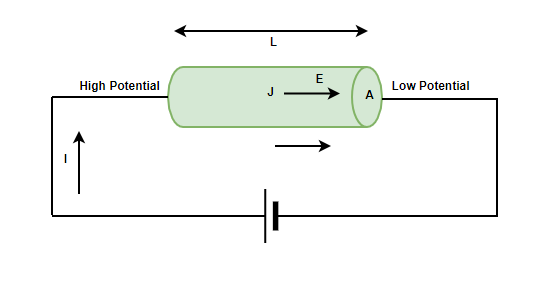GFG App
Open AppBrowser
Continue

# Electrical Resistance and Resistivity

One can think of many devices which are responsible for creating current in any given circuit. Generators, batteries, wall outlets, etc are necessary to maintain a particular electric current in the circuit. All such devices are essential for maintaining a potential difference across the circuit and are referred to as voltage sources. When a voltage source is connected across a conductor, it creates an electric field which causes the charges to move and this causes current. The values of the current that is generated strictly depends on the characteristic of the material. Any material opposes the flow of electric charge which is called resistance. It is developed due to resistivity which is a property of the material. Let’s see the concepts of resistance and resistivity in detail.

### Resistance

Water flowing through a pipe encounters resistance to its flow if the current is considered as water and the pipe is considered to be the conductor through which the current is flowing. The same analogy can be used to deduce the obstruction of flow in the case of current too. This obstruction of flow against the current is termed Resistance. The resistance in a wire experiencing a current I and voltage V is defined as,

R = V/I

Here, R denotes the resistance of the wire. Its unit is “ohms” which is denoted by Ω

Notice in the relation, the resistance depends inversely on the current flowing through the wire. Thus, the more the resistance of the wire, the less is the current flowing through it, which can also be deduced from the intuitive definition of the resistance.

### Resistance of a Wire

Picking the previous example of flowing water, one can draw the similarities between these two very different physical processes. The resistance offered to the water flowing through the pipe majorly depends on the length of the pipe and its cross-sections. In a similar way, the resistance offered by a conductor depends on the conductor’s size, shape, and material. The figure below shows a conductor which the length l, cross-sectional area A and resistivity (rho).The resistance of the conductor is given by,

R = ρl/A

Notice that, the resistance is proportional to the length of the conductor but inversely proportional to the cross-sectional area of the conductor.

### Resistivity

When a voltage is applied to a conductor, the electric field E is created inside it which pushes the charges to move. The current density that gets developed depends on the material and the electric field that is created. This density can be very complex but under reasonable assumptions, including assuming the metals at room temperature. This relation can be modeled using,

J = σE

Here, σ is the electrical conductivity.

Electrical conductivity is the measure of the material to conduct electricity. Conductors have more electrical conductivity than insulators. Conductivity is an intrinsic property of material, another intrinsic property of the material is the resistivity of electrical resistivity. It is a measure of how strongly a material opposes the flow of charges. It is denoted by the lower case greek letter rho. The electrical resistivity is reciprocal of the electrical conductivity.The unit of resistivity is given by ohm-meter(Ω-m).

### Sample Problems

Question 1: A battery of 5 Volts connected to a conductor induces a current of 10mA in the conductor. Find the resistance of the conductor.

The resistance of conductor is given by the relation,

R  = V/I

Given:

V = 5V

I = 10mA = 0.01 A

Plugging in the values inside the relation,

R = V/I

⇒ R = (5)/(0.01)

⇒ R= 500 Ohms.

Question 2: A battery of 20 Volts connected to a conductor induces a current of 50mA in the conductor. Find the resistance of the conductor.

The resistance of conductor is given by the relation,

R  = V/I

Given:

V = 20V

I = 50mA = 0.05 A

Plugging in the values inside the relation,

R = V/I

⇒ R = (20)/(0.05)

⇒ R= 400 Ohms.

Question 3: A battery of 100 Volts connected to a material induces a current of 0mA in the conductor. Find the resistance of the conductor.

The resistance of conductor is given by the relation,

R  = V/I

Given:

V = 20V

I = 0mA = 0 A

Plugging in the values inside the relation,

R = V/I

⇒ R = (20)/(0)

The resistance is closes to infinity, which means the material is an insulator.

Question 4: A cylindrical conductor of length 0.5m and cross-sectional area 0.01 m2. The resistivity of the material is 2 x 10-8 ohm-m. Find the resistance of the material.

The resistance of a conductor is given by,

R =Given

l = 0.5m

A = 0.01 m2

ρ = 2 x 10-8

Plugging the values in the relation given above,

R =⇒ R =⇒ R = 10-6

Question 5: A cylindrical conductor of length 2m and cross-sectional area 0.05 m2. The resistivity of the material is 4 x 10-8 ohm-m. Find the resistance of the material.

The resistance of a conductor is given by,

R =Given

l = 2m

A = 0.05 m2

ρ = 4 × 10-8

Plugging the values in the relation given above,

R =⇒ R =⇒ R = 1.6 × 10-6

My Personal Notes arrow_drop_up